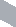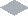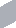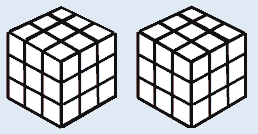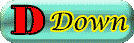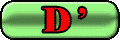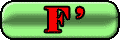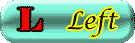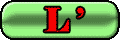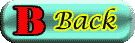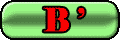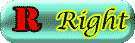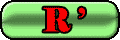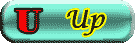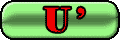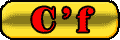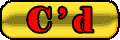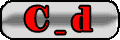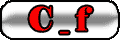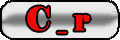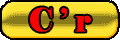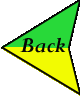Attention!
100 % work in IE 9.0 and Opera 12
.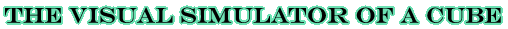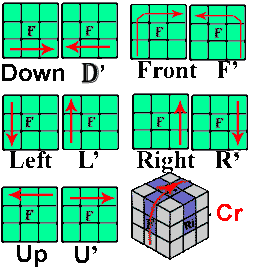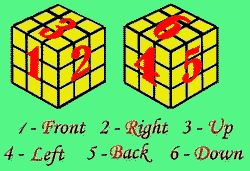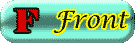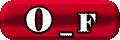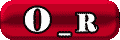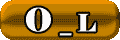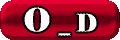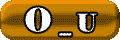F'=F_Opposite B'=Back_Opposite L'=Left_Opposite
R'=Right_Opposite U'=Up_Opposite D'=Down_Opposite
C'f=Center_Front_Opposite C'r=Center_Right_Opposite
Cl=(C ' r)=Cr*3 Cu=(C ' d) Cb=Cf
Of=O'b=[F B Cf] Or=[R L' Cr] Od=[D U' Cd] Ou=Od*3